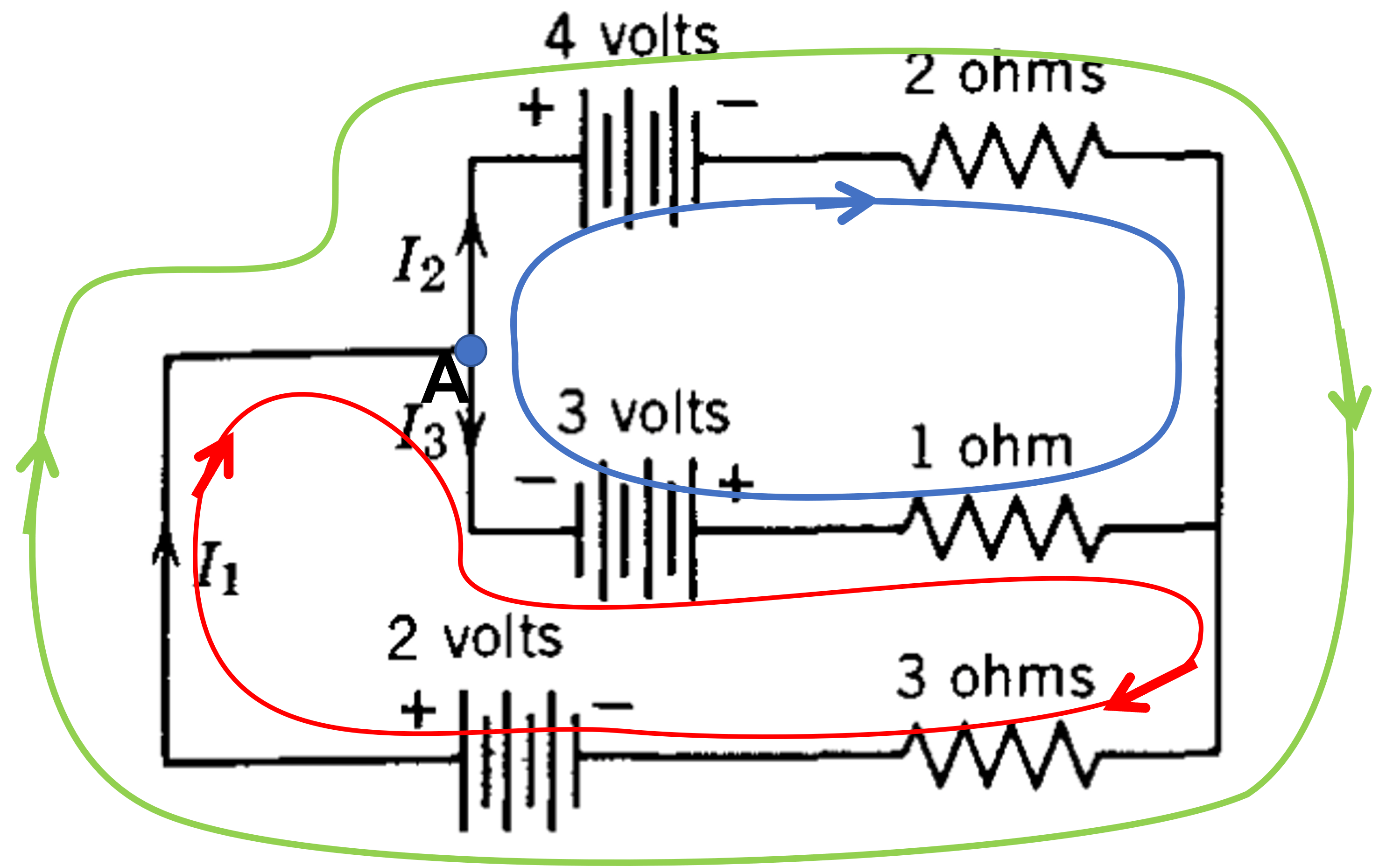# Matrix and Its Application for Solving Linear Equations Problems

Matrix is very useful in dealing with linear equations. There are a lot of branches in science where we deal with linear equation especially in physics.

In this post, we will take an example in physics where matrix will help us to solve the problems.

We will deal with simple electric circuits.

Let us take attention to the following figure.Find the currents I1, I2, and I3.

In solving electric circuit problems, we are equipped with Kirchhoff’s Laws, which is consist of two parts, namely:

1. Kirchhoff’s voltage law, and
2. Kirchhoff’s current law

Kirchhoff’s voltage law says that:

The algebraic sum of the changes in potential encountered in a complete traversal of any loop of a circuit must be zero.

Kirchhoff’s voltage law is also referred as Kirchhoff’s loop rule.

To apply this rule, first we freely choose the direction of the current in the circuit. Then, we mentally walk around the circuit until we are back at our starting point. The direction of walking does not have to be the same as the direction of the current. But for more convenience result, we encourage to choose the direction of walk to be same as the current’s direction.

Then we follow the rule below:

For a move through a resistance in the direction of the current, the change in potential is  -iR; in the opposite direction it is +iR.

For a move through an ideal emf device in the direction of the emf arrow, the change in potential is +ε; in the opposite direction it is -ε.

Meanwhile, the Kirchhoff’s current law says that:

The sum of the currents entering any junction must be equal to the sum of the currents leaving that junction.

Kirchhoff’s current law is also called Kirchhoff’s junction rule. It is simply a statement of conservation of charge for a steady flow of charge. It says that there is neither a buildup nor a depletion of charge at a junction.

Now, let us apply Kirchhoff’s laws above to the problem mentioned before.

For convenience, we will redraw the figure with addition of loops as below.We were asked to find three variables: I1, I2, and I3.

For 3 unknown variables, we must have 3 independent equations containing the three variables. To acquire the 3 equations, we choose three loops that lead us to three equations as below.

For red loop (lower loop), we can apply Kirchhoff’s voltage law as below:

We mentally start at positive sign of battery and walk in the direction as the arrow shown (clockwise). We found the following equation:

$$+ 3 – {I_3} – 3{I_1} + 2 = 0\,\,\,\, \Rightarrow \,\,\,\,{I_3} + 3{I_1} = 5\, \, \, …\, (1)$$

For the blue loop (upper loop), we found the following equation:

$$– 4 – 2{I_2} + {I_3} – 3 = 0\,\,\,\, \Rightarrow \,\,\,\,{I_3} – 2{I_2} = 7\,\,\, …\, (2)$$

For the green loop (the biggest loop), we could write the following equation:

$$– 4 – 2{I_2} – 3{I_1} + 2 = 0\,\,\, \Rightarrow \,\,\,2{I_2} + 3{I_1} = – 2\,\,\, …\, (3)$$

Now we apply Kirchhoff’s current law at point A to get the following equation:

$${I_1} – {I_2} – {I_3} = 0\,\, \Rightarrow \,\,{I_1} = {I_2} + {I_3}\,\,\, … \, (4)$$

Insert equation (4) into equation (1) so we found the following equations:

$${I_3} + 3\left( {{I_2} + {I_3}} \right) = 5\,\,\, \Rightarrow \,\,\,\,3{I_2} + 4{I_3} = 5\,\,\, … \, (5)$$

Now, let us collect the equations the we will use to proceed (eq. (2), (3) and (5)) as below

$$– 2{I_2} + {I_3} = 7\,\,\, … \, (2)$$

$$3{I_1} + 2{I_2} = – 2\,\,\, … \, (3)$$

$$3{I_2} + 4{I_3} = 5\,\,\, … \, (5)$$

If we transform the equations to be matrix, we can solve the variables by using row-reduction.

Let us do it.

The matrix for row-reduction of the group of equations above is

$$\begin{pmatrix} 0 & -2 & 1 & 7 \\ 3 & 2 & 0 & -2 \\ 0 & 3 & 4 & 5 \end{pmatrix}$$

Now we do row-reduction:

1. Interchange row 1 with row 2.

$$\begin{pmatrix} 0 & -2 & 1 & 7 \\ 3 & 2 & 0 & -2 \\ 0 & 3 & 4 & 5 \end{pmatrix} \underrightarrow{R1<->R2} \begin{pmatrix} 3 & 2 & 0 & -2 \\ 0 & -2 & 1 & 7 \\ 0 & 3 & 4 & 5 \end{pmatrix}$$

1. Multiply row 2 with 3, row 3 with 2 and add row 2 (after multiplied) with row 3 after multiplied.

$$\begin{pmatrix} 3 & 2 & 0 & -2 \\ 0 & -2 & 1 & 7 \\ 0 & 3 & 4 & 5 \end{pmatrix} \underrightarrow{2R3+3R2} \begin{pmatrix} 3 & 2 & 0 & -2 \\ 0 & -2 & 1 & 7 \\ 0 & 0 & 11 & 31 \end{pmatrix}$$

From the last result we found that

$$11{I_3} = 31\,\, \Rightarrow \,\,{I_3} = \frac{{31}}{{11}}$$

$$– 2{I_2} + {I_3} = 7$$

Substitute I3 = 31/11 into the equation above we find:

$$– 2{I_2} + \frac{{31}}{{11}} = 7\,\, \Rightarrow \,\, – 2{I_2} = \frac{{77}}{{11}} – \frac{{31}}{{11}} = \frac{{46}}{{11}}\,\,\, \Rightarrow \,\,\,{I_2} = – \frac{{46}}{{22}} = – \frac{{23}}{{11}}$$

From row 1 of the last result give us

$$3{I_1} + 2{I_2} = – 2$$

Inserting I2 = -23/11 will give us

$$3{I_1} + 2\left( { – \frac{{23}}{{11}}} \right) = – 2\,\, \Rightarrow \,\,3{I_1} = \frac{{46}}{{11}} – \frac{{22}}{{11}} = \frac{{24}}{{11}}$$

Or ${I_1} = \frac{8}{{11}}$

Thus, we found I1 = 8/11, I2 = 23/11, and I3 = 31/11.

The minus sign in I2 indicates that the direction of I2 is actually opposite with the direction that we have drawn in the figure.